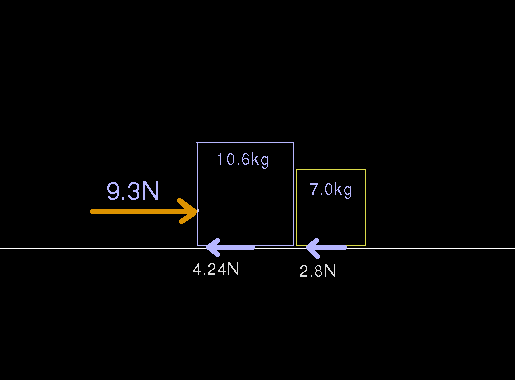# Magnitude of contact forces

## Homework Statement

Alex is asked to move two boxes of books in contact with each other and resting on a rough floor. He decides to move them at the same time by pushing on box A with a horizontal pushing force FP = 9.3 N. Here A has a mass mA = 10.6 kg and B has a mass mB = 7.0 kg. The contact force between the two boxes is Fc
The coefficient of kinetic friction between the boxes and the floor is 0.04. (Assume fp acts in the +x direction)
a) What is the force exerted on mB by mA? In other words what is the magnitude of the contact force
(c) If Alex were to push from the other side on the 7.0-kg box, what would the new magnitude of

## Homework Equations

A=Fp-f/M

3. The 0
attempt at a solution

contact force =force-friction-ma

Friction= (0.04)(17.6*9.8)

Last edited by a moderator:

NascentOxygen
Staff Emeritus
Hi CristinaCotto. http://img96.imageshack.us/img96/5725/red5e5etimes5e5e45e5e25.gif [Broken]

That is the friction force. When determining the contact force, I think a free body diagram is essential. Your equation looks promising.

Last edited by a moderator:
Thank you, and i haven't grasped the concept of free body diagrams yet.
I tried the equation on a problem my tutor helped me with but i didn't get the same answer, What could be my fault?
if i get 9.3-6.9(7.0 * .136)= 1.448N
is this correct?

NascentOxygen
Staff Emeritus
Thank you, and i haven't grasped the concept of free body diagrams yet.
I tried the equation on a problem my tutor helped me with but i didn't get the same answer, What could be my fault?
if i get 9.3-6.9(7.0 * .136)= 1.448N
is this correct?
You need to explain where these numbers appeared from and what equation you are using.

to get a number for friction i did : (0.4)(17.6*9.8)=68.992
0.4 being kinetic friction, 17.6 is total mass and 9.8 is gravity. 9.3 is pushing force fp
total forces= 0.04(9.8)(17.6)=.136
A= fp-f/m
a=9.3-.136/17.6=.521m/s^2
contact force =force-friction-ma
9.3-.136(7.0*.521)=5.517N
Im so sorry i confused my numbers, its actually this but 7.0 is the mass of box b.

NascentOxygen
Staff Emeritus
to get a number for friction i did : (0.4)(17.6*9.8)=68.992
0.4 being kinetic friction, 17.6 is total mass and 9.8 is gravity. 9.3 is pushing force fp
total forces= 0.04(9.8)(17.6)=.136
A= fp-f/m
a=9.3-.136/17.6=.521m/s^2
contact force =force-friction-ma
9.3-.136(7.0*.521)=5.517N
Im so sorry i confused my numbers, its actually this but 7.0 is the mass of box b.
I think you probably have the right idea, but this post is badly in need of proof-reading! I can see a wrong value, missing brackets, and a missing sign. These need fixing.

He is pushing on the 10.6 kg box, right?

I am so sorry, I did it how I saw it on a question on a different website.
Yes the pushing force is on the 10.6

NascentOxygen
Staff Emeritus
I see in another post that you are attempting physics without having the prerequisites, so let's see if we can sort out some of your mistakes here. You can't afford to let misunderstandings slip by at this early stage.

A diagram is essential. I have marked in only the horizontal forces to avoid clutter.(You have confused me by using both 0.4 and 0.04 as the co-efficient of friction. I have to use some value, so shall assume it's 0.4)

The applied force must overcome friction, and any remaining force will accelerate the combined blocks according to the equation F = m.a

i.e., applied force = friction + 17.6kg x acceleration
so acceleration = (9.3N - 7.0N) / 17.6 = 0.136 m/s2

You have that figure in your working, so you got that figured out.

If there were no friction, then the force needed on the 7kg block to give it an acceleration of 0.136 m/s2 would be:
F = m.a = 7.0 x 0.136 = 0.952N

But the block also has 2.8N of friction to be overcome, so to move the 7kg block the required force acting on it is:
= 0.952N + 2.8N
= 3.8N

Since he is pushing on the 10.6kg block, it in turn must be pushing on the 7kg block with a contact force
= 3.8N

Part (c) of the problem asks what would the force of one block on the other be if he were to push on the 7kg block instead. Can you answer that?

Last edited:
NascentOxygen
Staff Emeritus